# We Helped With This MATLAB Programming Homework: Have A Similar One?

SOLVEDCategory Programming MATLAB College Solved Pay For Matlab Homework

## Short Assignment Requirements

the project file is attached below.All intructions are mentioned in attached file(project).How to do the problem,everything is in the file.I f you are capable to do this assignment please inform me and in case you need information of lab section, my name and student number I will write it because it asks for it. #(number)=50245072 Name Surname: Baris Cetinok Lab section:B4

## Assignment Description

EAS-230 Fall 2017

Programming Project # 1 (PP1)

Score:               /100

Directions:

1.  Your three m-files must be named as in the deliverables section of this assignment.

2.  All three m-files (two scripts and one function) must be uploaded to UBlearns before 11:59 PM on Monday, Nov. 13, 2017 (Please do not upload files ending in “.m~”, “.sav” or “.mat”.) A pdf copy of the report (part 3) must also be uploaded to UBlearns on the same due date.

3.  A paper copy (the report) including your codes, the results and analysis must also be written (a template of the report will be posted on UBlearns). Your report must be turned-in at the start of your first lecture of the week of Monday, Nov. 13, 2017 (Mon or Tue, depending on your section). Be sure to write your name, person # and lab section on every page.

4.  You must write your own code and follow all instructions to get full credit. You are not allowed to use codes or scripts found on the internet or any other references.

5.  You must use good programming practices, including indentation, commenting your functions scripts and choosing meaningful variable names to make your programs self-documenting.

6.It is your responsibility to make sure that your functions/scripts work properly and are free of errors by utilizing the resources at your disposal.

EAS 230 – Fall 2017 – PP1                                                                                             Page 1 of 8

Background, :

The friction factor in fully developed turbulent pipe flow depends on the Reynolds number and the relative roughness 𝜀/𝐷, which is the ratio of the mean height of roughness of the pipe to the pipe diameter. The functional form of this dependence cannot be obtained from a theoretical analysis, and all available results are obtained from painstaking experiments using artificially roughened surfaces (usually by gluing sand grains of a known size on the inner surfaces of the pipes). Most such experiments were conducted by Prandtl’s student, J. Nikuradse in 1933, followed by the works of others. The friction factor was calculated from the measurements of the flow rate and the pressure drop. The experimental results obtained are presented in tabular, graphical, and functional forms obtained by curve-fitting experimental data. In 1939, Cyril F. Colebrook (1910 –1997) combined the available data for transition and turbulent flow in smooth as well as rough pipes into the following implicit relation known as the Colebrook equation

1                                    𝜀/𝐷          2.51= −2.0 log (       +           )                                                                         (1)

√𝑓                                    3.7         Re √𝑓

This equation is only applicable for turbulent flow, Re ≥ 4000. For laminar and transitional flow, Re < 4000, the friction coefficient can be determined as

64

𝑓 =(2)

Re

We must note that the logarithm in Equation (1) is a base 10 rather than a natural logarithm. In 1942, the American engineer Hunter Rouse (1906 –1996) verified Colebrook’s equation and produced a graphical plot of f as a function of ε and the product Re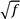. He also presented the laminar flow relation and a table of commercial pipe roughness. Two years later, Lewis F. Moody (1880 –1953) redrew Rouse’s diagram into the form commonly used today.

The now famous Moody chart is shown in Figure 1. It presents the Darcy friction factor for pipe flow as a function of the Reynolds number and 𝜀/𝐷 over a wide range. It is probably one of the most widely accepted and used charts in engineering.

At very large Reynolds numbers (to the right of the dashed line on the chart) the friction factor curves corresponding to specified relative roughness curves are nearly horizontal, and thus the friction factors are independent of the Reynolds number (Fig. 1 ). The flow in that region is called fully rough turbulent flow or just fully rough flow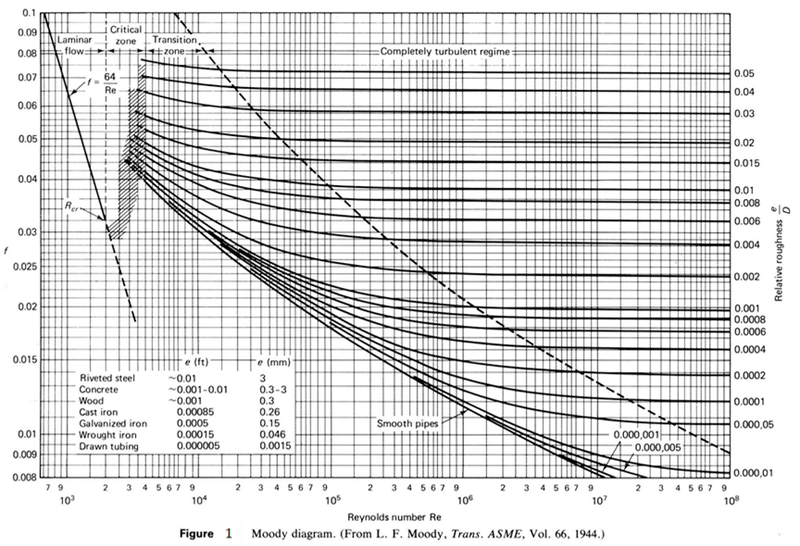EAS 230 – Fall 2017 – PP1                                                      Page 3 of 8

Deliverables:

You are required to reproduce the friction coefficient, f , as a function of Reynolds number, Re, for laminar flow and as a function of Re and the relative roughness, 𝜺/𝑫, for turbulent flow. Your data must be presented in both Table format and chart format (Moody chart shown in Fig.1) by doing the following:

1. (30 pts) Create a script file named FCLT_UBITName.m to tabulate the value of the friction coefficient for both laminar and turbulent flows. _UBITName is your UBITName as in your email UBITName@ buffalo.edu.

a.       Create a variable RelRough and assign to it all values of the dimensionless roughness 𝜺/𝑫

𝜺          𝜺 shown in Figure 2 starting from= 𝟎 , for smooth pipes, till             = 𝟎.05. The element values

𝑫                                                                                     𝑫

of the vector RelRough must be exactly the same as those values shown in Figure 2 next to each curve (see the values inside the dashed rectangle).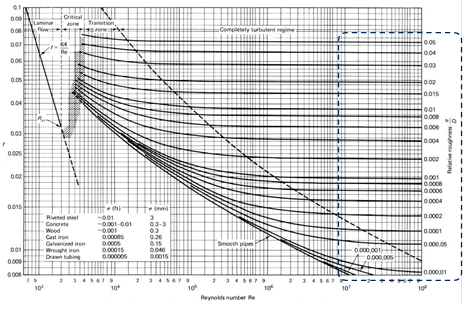Figure 2: Values of the relative roughness in Figure 1.

b.       Your script must determine the value of the friction coefficient f  (FC variable in MATLAB) as a function of Re for every element of the vector RelRough. Noting that for laminar flow, the friction coefficient f is a function of Re only while for turbulent flow, it is a strong function of both Re and 𝜺/𝑫. You must create a variable Re that represents the x-axis in Figure 1, noting that the Re axis is logarithmic. For 600 < 𝑅𝑒 < 4000 , you can assume laminar flow and the friction coefficient FC  can be determined from equation (2) as a function of Reynolds number only. Start with a value of the laminar flow Reynolds number ReL of 600 and increase it constantly by 100 to 3900. You can assign your friction factor values for laminar flow to a variable called  FCL. You must note that FCL is a vector (function of Re only).

c.        For turbulent flow (Re ≥ 4000), Equation (2) must be used to determine the turbulent friction coefficient f (FCT).  You must note that f  is implicit and appears in both sides of Equation (1). Accordingly, to determine the friction coefficient f  numerically, an iterative technique must be developed. You must also note that f for turbulent flow is a function of both Re and  𝜺/𝑫. Create a new variable ReT = [4000 5000 6000 … 10000 20000  30000 … 100000 200000 300000 … 1000000…] and follow this pattern until 108. Use good programming practices and Do Not write the elements of the variable explicitly. For every value of RelRough, FCT must be determined for all the values of ReT. You must note that FCL is a vector (function of Re only) while FCT is a matrix (function of Re and 𝜀/𝐷).

d.       To develop the iterative technique for determining the turbulent friction coefficient, Equation (1) can be rewritten as

𝑓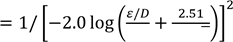(3)

3.7         Re √𝑓

You can start by entering a guess value for f (Use 𝑓 = 1 as a first guess), and accordingly the right hand side can be determined and the result will be assigned to the variable f in the left hand side. You can use variable names of FCT_guess or FCT_old for the guessed value of f and FCT_cal or FCT_new for the calculated value of f.   The function should then compare between the guessed value of f and the calculated value of f. If they are close to each other by a specific tolerance (1E-14), the iteration must stop and the calculated value of f must be saved. Otherwise, the iteration procedure must continue taking the calculated value from the previous iteration as the new guess value of the current iteration and continue as before. In order to avoid infinite loop in case of divergence, you must set a maximum number of iterations to 30. If this number was reached the function should stop and display a message “The program failed to converge in the allowable number of iterations”.

e. After calculating the friction coefficient for both laminar and turbulent flow, you must display your data in Table format, as shown below, for:

i.        Laminar flow

ii.      Turbulent flow

^^^^^^^^^^^^^^^^^^^^^^^^^^^^^^^^^^^^^^^^^^^^^^^^^^^^^^^^^^^^^^^

Friction Coefficient for Laminar Flow in pipes*1000

(Re < 4000)

------------------------------------------------

Reynolds #    |  Friction Coefficient

------------------------------------------------

600             |    106.66667

-------------------------------------------------

700             |     91.42857

-------------------------------------------------     …………………………………………. ^^^^^^^^^^^^^^^^^^^^^^^^^^^^^^^^^^^^^^^^^^^^^^^^^^^^^^^^^^^^^^^^

Friction Coefficient for Turbulent Flow in pipes*1000

(Re >= 4000)

------------------------------------------------

Reynolds# |  Relative Roughness

------------------------------------------------

### |  0         |   1E-6 |  5E-6 |  ---

------------------------------------------------

4000          |   ---- |  ---- |  ---- |  ---

-------------------------------------------------

5000          |   ---- |  ---- |  ---- |  ---

------------------------------------------------- ………………………………………….

Display only 5 values of the relative roughness per page. You must notice that the values of the friction coefficient to be displayed in the table for both laminar and turbulent flow must be the values calculated from your script after being multiplied by 1000 for better display.

2.       (20 pts) Use the techniques you developed before for determining the friction coefficient for laminar and turbulent flow in a User Defined Function (UDF) called fcoeff_UBITName.m. The function must take Re and 𝜺/𝑫 as input arguments and returns the friction coefficient f for either laminar or turbulent flow as the output argument. Note that fcoeff_UBITName.m  will use Equation (2) for the laminar friction coefficient and the iterative technique developed in part 1.d for determining the turbulent friction coefficient from Equation (1).

3.       (50 pts)

a.       (30 pts) You are now required to reproduce Figure 1 (the Moody chart) with all of its details using the function fcoeff_UBITName(). You must write a new script file called

MoodyCh_UBITName.m, which may be a modification of FCLT_UBITName.m script you developed for part 1. Use only 8 points for the laminar flow Reynolds number ReL =  [600 700 800 900 1000 2000 3000 3900] for the plot of the laminar flow line in the Figure. Use good programming practice and Do Not write the elements explicitly. For turbulent flow, use the same values for vector RelRough you have developed for FCLT_UBITName.m script. For turbulent Reynolds number, ReT, you must assigned new values that develop a smooth curve. You can use the function logspace() (see the help) with 1000 elements or more to cover all the range of turbulent Reynold number 4000 ≤ Re𝑇 ≤ 1 × 108. You must note that logspace() starts with 103 in this range. For calculating the friction factor either for laminar flow or for turbulent flow, your script MoodyCh_UBITName.m must use(call) the function fcoeff_UBITName() to determine the friction coefficient for every value of Re and 𝜺/𝑫. You can assign your friction factor values for laminar flow to a variable called  FCL and the friction factor values for turbulent flow to a variable called  FCT, as before. After creating all the variables, create your plot using the appropriate plotting function. You must note that, if you are plotting x vs. Y where x is a vector and Y is a matrix (m×n), the length of x must be equal to the number of rows of Y, (m), and MATLAB will be plotting x vs. Y column by column, i.e., (x, C1Y), (x, C2Y), …, (x, CnY). You can develop your figure in color using line width of 1. You must annotate your figure to be as close as to the Moody chart in Figure 1 with all the details such as the axes labels, the axes limits, the tic labels, etc. You must add texts for the 𝜀/𝐷 of every curve. The figure caption, as a text, must also be shown, as well as, the table of roughness for various materials.

b.       (20 pts) The dashed line that determines the start of the fully rough turbulent flow region (see the red dashed line in Fig. 3) can be plotted once its intersection points (the red crosses in Fig. 3), with all relative roughness 𝜺/𝑫 curves, starting from 0.00005 to 0.05, are determined. In order to numerically do this in your script MoodyCh_UBITName.m, you are first required to produce smooth curves for every value of 𝜺/𝑫 by using enough points (≥ 1000 on log Re scale) and then scan every curve to determine the point at which the curve starts to be almost horizontal (not a function of Re). You can do that by determining the relative change in f as

|𝑓2−𝑓1|

(4)

𝑓1

Once this change is less than a certain tolerance (10-4), you can assume that the curve is horizontal and you must determine the coordinate of this point (Re, f) for every curve of 𝜺/𝑫. The line connecting these points will be the dashed line in Figure 1 and you are required to add this graph to your plot using line width of 2.

References:

 Y. Cengel and J. Cimbala, “Fluid Mechanics Fundamentals and Applications,” 2nd edition, McGrawHill, 2010.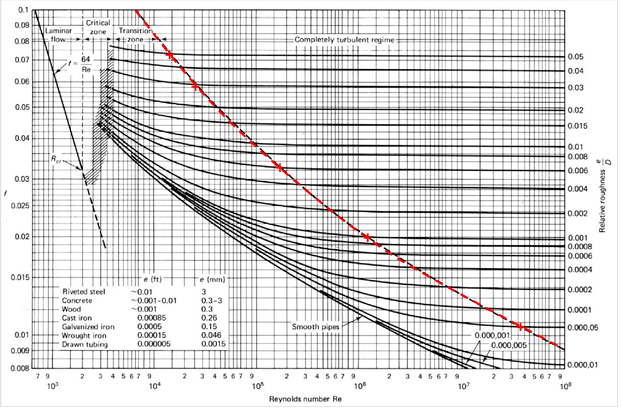Figure 3: The start of the completely rough turbulent region.

Is it free to get my assignment evaluated?

Yes. No hidden fees. You pay for the solution only, and all the explanations about how to run it are included in the price. It takes up to 24 hours to get a quote from an expert. In some cases, we can help you faster if an expert is available, but you should always order in advance to avoid the risks. You can place a new order here.

How much does it cost?

The cost depends on many factors: how far away the deadline is, how hard/big the task is, if it is code only or a report, etc. We try to give rough estimates here, but it is just for orientation (in USD):

 Regular homework \$20 - \$150 Advanced homework \$100 - \$300 Group project or a report \$200 - \$500 Mid-term or final project \$200 - \$800 Live exam help \$100 - \$300 Full thesis \$1000 - \$3000

How do I pay?

Credit card or PayPal. You don't need to create/have a Payal account in order to pay by a credit card. Paypal offers you "buyer's protection" in case of any issues.

Why do I need to pay in advance?

We have no way to request money after we send you the solution. PayPal works as a middleman, which protects you in case of any disputes, so you should feel safe paying using PayPal.

Do you do essays?

No, unless it is a data analysis essay or report. This is because essays are very personal and it is easy to see when they are written by another person. This is not the case with math and programming.

Why there are no discounts?

It is because we don't want to lie - in such services no discount can be set in advance because we set the price knowing that there is a discount. For example, if we wanted to ask for \$100, we could tell that the price is \$200 and because you are special, we can do a 50% discount. It is the way all scam websites operate. We set honest prices instead, so there is no need for fake discounts.

Do you do live tutoring?

No, it is simply not how we operate. How often do you meet a great programmer who is also a great speaker? Rarely. It is why we encourage our experts to write down explanations instead of having a live call. It is often enough to get you started - analyzing and running the solutions is a big part of learning.

What happens if I am not satisfied with the solution?

Another expert will review the task, and if your claim is reasonable - we refund the payment and often block the freelancer from our platform. Because we are so harsh with our experts - the ones working with us are very trustworthy to deliver high-quality assignment solutions on time.

## Popular Solved Assignments Like This

Customer Feedback

"Thanks for explanations after the assignment was already completed... Emily is such a nice tutor! "

Order #13073